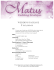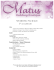# Understanding the development of the proving process within a

## Transcription

Understanding the development of the proving process within a
```Danh Nam NGUYEN, Universität Würzburg
Understanding the development of the proving process within
a dynamic geometry environment
Abstract. In this paper we investigated the development of the proving
process within a dynamic geometry environment in order to provide
tertiary students with a strategy for proving. As a result, we classified
different levels of proving and designed an interactive help system
corresponding with these levels. This help system makes a contribution to
bridge the cognitive and structural gaps between conjecture and proof. We
also propose three basic conditions for understanding the development of
the proving process.
Keywords. Proving process, level of proving, help system, abduction.
1. Introduction
In this research we consider proof as the final product of the proving
process. Therefore, understanding the development of the proving process
contributes in gaining insight into the invention of mathematical ideas and
the difficulties in constructing proofs. That is also the reason why tertiary
students should learn how to write, read, understand, and construct proofs.
To support students in learning proofs, we provided them a methodological
model with seven levels of proving and built the interactive help system
based on this model (see Fig. 1). This help system contains open-ended
questions and explorative tasks with two functions: to direct thought and to
convey information. An open-ended question was used to help students
look for geometric invariants and combine valid arguments into a formal
proof. An explorative task was used to help students explore the problem
on their own. During students’ proving process, by answering open-ended
questions as well as tackling explorative tasks, the idea of proofs may
emerge gradually and arguments are produced as well.
2. Methodology
The proving process is a sequence of mental and physical actions, such as
writing or thinking a line of a proof, drawing or visualizing a diagram,
producing arguments, etc. Therefore, we classified seven levels of proving
that represent the developmental phases in the proving process. These
levels are described as follows: level 0 (information) provides students with
clear information aimed at pointing out the principal parts of the problem,
the unknown, the data, and the conclusion; level 1 (construction) guides
6
Interactive Help System
5
4
3
Open-ended
question
Explorative
task
2
1
0
Delving
Proof
Argumentation
Conjecture
Invariance
Construction
Information
The development of the proving process
students to model and construct the figures in a dynamic geometry
environment; level 2 (invariance) guides students to search for geometric
invariants that support in generating the ideas for proofs; level 3
(conjecture) supports students in formulating conjectures that often
originate from experimental activities; level 4 (argumentation) guides
students to produce arguments by explaining ‘observed facts’ and
validating formulated conjectures; level 5 (proof) guides students to write
proofs based on produced arguments; level 6 (delving) suggests students to
delve into the problem such as generalization, specialization, analogy, etc.
Fig. 1 A methodological model for understanding the proving process
The data of the empirical research was collected during the summer
semester 2010/2011. The students were enrolled in a required elementary
geometry classes for a teacher training course and divided into groups of
three who sat together at one computer. We also installed Wink software
on each computer in order to capture and audio-record of all the working
worksheets and group discussions. In this research, we also used Toulmin
model in order to analyze the structure of argumentation during students’
discussion in their group. According to this model, in any argumentation
the first step is expressed by a claim (C) such as an assertion, an opinion or
a conjecture. The second step consists of the production of data (D)
supporting the claim. The warrant (W) can be expressed as a principle, a
rule or a theorem for supporting for the data-claim relationships (see
Toulmin, 1958). This model is not only useful to represent a deductive step
but also a powerful tool to represent an abductive structure, which can be
used to explicate the role of abduction in transition from conjecturing to
proving modality (see e.g. Pedemonte & Reid, 2011). The following model
describes the way students finding data (?) for validating their claim when
they know one rule for supporting the claim:
Fig. 2 Abduction in Toulmin model of argumentation
3. Understanding the development of the proving process
In this paper we provide three basic conditions for understanding the
development of the proving process: (1) Realizing geometric invariants for
generating ideas for proofs. This phase supports students in getting more
data for proving and searching geometric invariants for generating the ideas
of proofs. (2) Constructing a cognitive unity in the transition from
conjecture to proof. This process produces arguments for validating
conjectures and writing proofs. In other words, cognitive unity is a
phenomenon where some arguments, which are produced for the
plausibility of the conjecture and become ingredients for the construction
of a proof (Boero et al., 1996). (3) Organizing arguments in order to write
a formal proof. This is one of the most difficult phases in the proving
process because students need to organize (select and combine) produced
arguments as a chain of logical valid arguments for writing proofs.
We chose the discussion of one typical group, which was audio-recorded
by using Wink software, to analyze students’ arguments during proving
process through the following problem: A river has straight parallel sides
and cities A and B lie on opposite sides of the river. Where should we build
a bridge in order to minimize the traveling distance between A and B (a
bridge, of course, must be perpendicular to the sides of the river)? The
interactive help system provided students with some open-ended questions
and explorative tasks like “What is relationship between two lines AD and
EB when the length of the broken line ADEB is minimal?”, “Compare the
length of the broken line ADEB and the length of the broken line AGHB”,
and so on. Firstly students realized a key geometric invariant by using
GeoGebra software: “the line AD is an image of the line EB under a
translation in the vector ⃗⃗⃗⃗⃗ direction when the length of the broken line
ADEB is minimal”. Then they determined two points G and H which are
best places for building the bridge and some spontaneous arguments were
also produced for validating this conjecture. The following dialogue was
extracted from three-student group’s discussion and Toulmin model was
used to represent the structure of argumentation:
 Student 1: It is obvious that the length of the broken line AGHB is smaller the length
of the broken line ADEB. How can we prove this inequality when we have the following
data ED = HG = BB’, HB = GB’, EB = DB’?
D1 = ?
C1: AG + GH + HB ≤ AD + DE + EB
(1)
W1: ED = HG = BB’, HB = GB’, EB = DB’
 Student 2: We may consider the inequality AG + GB’ + B’B ≤ AD + DB’ + B’B (2)
D2 = ?
C2: AG + GB’ + B’B ≤ AD + DB’ + B’B
W2: BB’ is common summand
 Student 3: Look at the inequality! We have
BB’ as a common summand and three points A,
G, B’ are collinear. Therefore, we need to prove
that AG + GB’ = AB’ ≤ AD + DB’.
D3 = ?
C3: AB’ ≤ AD + DB’ (3)
W3: Triangle inequality (ADB’)
In order to write a formal proof, students
followed a sequence of the inequalities
(3)  (2)  (1). Therefore, by using Toulmin model, we interpret that
students always reverse ‘abductive structure’ so that they can find the data
for validating the claims, produce arguments, and write a formal proof.
4. Conclusions
This paper proposes a methodological model and three basic conditions for
understanding the development of the proving process within a dynamic
geometry environment. This model also provides tertiary students with
appropriate strategies and tools as a means of exploration, discovery, and
invention. The interactive help system can support students in realizing
geometric invariants, producing arguments, and writing a formal proof. The
findings of this research also provide mathematics teachers with a strategy
for teaching proof and the proving process at the tertiary level.
References
Boero, P., Garuti, R., & Mariotti, M. A. (1996): Some dynamic mental processes
underlying producing and proving conjectures. Proceedings of the 20th PME
International Conference, Valencia, Spain, vol.02, pp.121-128.
Pedemonte, B., & Reid, D. (2011): The role of abduction in proving processes.
Educational Studies in Mathematics, 76, 281-303.
Toulmin, S. (1958): The uses of argument. Cambridge: Cambridge University Press.
```

### WEDDING PACKAGE - The BestMore information

### WEDDING PACKAGE - The BestMore information